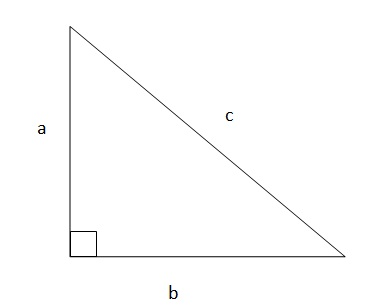# Geometry Problems

 Categories : Geometry Problems Drawing diagrams accurately in GMAT Geometry questions will allow you to capture the essential information from the Question. It’s not always easy. Here is a hands-on Experience for you: Draw the diagram as your read the question(below) and then go through the analysis.Q) A circular table consists of a glass center surrounded by a metal ring of uniform width. If the metal ring has a width of 2 inches, and the glass center has a diameter of 4x inches, what fraction of the table’s surface is made up by the metal ring, in terms of x?There’s no underhanded trick in this question, nor is there anything super complicated to incorporate into your diagram. But you should always be very mindful of the details of the question while drawing your diagram, since after you do so, you’re less likely to look at the information given in the problem. Indeed, it’s a waste of time to do so, since the information is presented much more usefully in your diagram! But this also means that if you make a mistake in the diagram, you may not correct it – and it’s very frustrating to get a problem wrong simply because your diagram was drawn incorrectly.If it helps you to think figuratively, consider this metaphor I’ve *ahem*... Categories : Area, Geometry Problems Rhombus ABCD below is divided into three areas with AGH = 1/3rd Area of ABCD, ECF = 1/5th Area of ABCD. What is the ratio of Area (AGH) to CD, given that AC=12 and BD =16?a) 10/3b) 11/3c) 13/3d) 17/3e) 19/3SolutionTo solve this question, you have to know the formula to find the area of a Rhombus. The most common formulas and properties to remember in a Rhombus are1) Area = base x altitude2) Altitude = ½ x sqrt (diagonal1^2 + diagonal2^2)3) Area = (diagonal1 X diagonal2)/24) All the sides are equal in a Rhombus... Categories : Geometry Problems Let us try to tackle the problem of Special Right Triangles and Pythagorean Triplets, today. The theory will be followed by suitable examples for special right triangles. As you must be aware, the Pythagorean Theorem is one of the most used tools in geometry.  Its three main features are: 1) One of the angles is a right angle2) The sides which are adjacent to the right angle are termed as the base and perpendicular/height 3) The third side is known as the hypotenuseFor the figure given below, the hypotenuse is ‘c’, the base is ‘b’ and the perpendicular or height is ‘a’.  As proposed by Pythagoras, the sum of square of sides adjacent to right angle is equal to the square of the third side, better known as the hypotenuse. Putting these words into equation form, we see:                      a^2+ b^2=c^2Two major takeaways from this theorem are:a) It is applicable only to right angled trianglesb) The choice of base and perpendicular is irrelevant as far as the two sides adjacent to the right angled... Categories : Data Sufficiency, Geometry Problems, Problem Solving A sizeable number of GMAT math test questions belong to the Geometry section.  Some of these questions test  a candidate’s ability to understand 2-Dimensional Geometry by asking the candidate to calculate the area, perimeter or circumference of a geometrical shape. The following geometrical shapes are most common – Triangles, Quadrilaterals, Rectangles, Rhombuses, Squares, Circles and Trapeziums. Triangles – A triangle represents an enclosed shape made by joining three straight lines. The area of a triangle can be calculated as follows: Area = ½*Base Side*Height of the triangleIn this formula, the Base Side can be any side of the triangle. However, depending on the base side chosen, height of the triangle needs to be ascertained. Height of the triangle is the shortest perpendicular distance from the Base side to the height of the Apex of that triangle.  Note that the height of a triangle may need to be calculated outside the triangle, depending on the base side chosen.... Categories : Geometry Problems Ashley and Bill simultaneously begin traveling around a circular road. Both start from the same point. Ashley travels counterclockwise and Bill travels clockwise. The two first meet after Ashley travels 40 miles per hour for 2.5 hours and Bill travels 6.4 miles per hour for 4 hours. What is the approximate diameter of the circular road (in miles)? Use 3.14 for ?.A. 20B. 40C. 46.4D. 116E. 125.6AnswerTo visualize this question, a circle is just a line segment joined at its two ends. Lets first cut the circle at a the starting point and make it in to a straight line. Notice that cars A and B are at opposite ends of the line traveling towards each other. After 2.5 hours, and at a rate of 40 mph, Car A has traveled 100 miles east. Car B has traveled 4 hours at 6.4 miles: 25.6 miles. To determine the diameter we simply plug in to the formula C = ? D where D is the diameter and C is the circumference and ? is about 3.14. We can take C to equal 126 and ? to equal 3.14. The diameter is therefore equal to: D = C/?= 126/3.14 equals approximately 40.Correct Answer - Choice B

Get F1GMAT's Newsletters (Best in the Industry)

• Ranking Analysis
• Post-MBA Salary Trends
• Post-MBA Job Function & Industry Analysis
• Post-MBA City Review
• MBA Application Essay Tips
• School Specific Essay Tips
• GMAT Preparation Tips